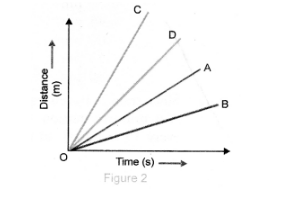# Four cars A, B, C and D are moving on a levelled road.`
Question:

Four cars $A, B, C$ and $D$ are moving on a levelled road. Their distance versus time graphs are shown in Fig. 2 Choose the correct statement $Q$(a) Car A is faster than car D

(b) Car B is the slowest

(c) Car $D$ is faster than car $C$

(d) Car $\mathrm{C}$ is the slowest.

Solution:

(b) Explanation: Slope of distance - time graph = speed of object.